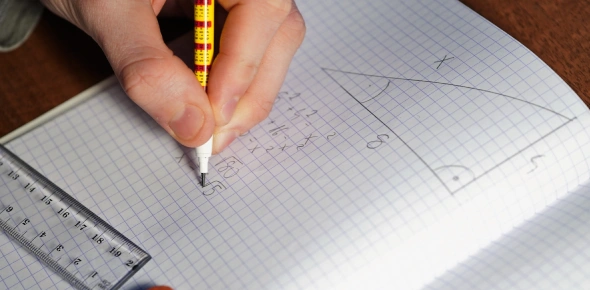# Area Of Triangles

Approved & Edited by ProProfs Editorial Team
The editorial team at ProProfs Quizzes consists of a select group of subject experts, trivia writers, and quiz masters who have authored over 10,000 quizzes taken by more than 100 million users. This team includes our in-house seasoned quiz moderators and subject matter experts. Our editorial experts, spread across the world, are rigorously trained using our comprehensive guidelines to ensure that you receive the highest quality quizzes.
| By Alohamom
A
Alohamom
Community Contributor
Quizzes Created: 1 | Total Attempts: 4,885
Questions: 6 | Attempts: 4,909SettingsTake the assigned math quiz. This will be a good practice for your quiz in class.

• 1.

### 1.  What does the letter b stand for in the formula  A = bh

• A.

Area

• B.

Base

• C.

Height

• D.

Length

B. Base
Explanation
In the formula A = bh, the letter b represents the base. The base is the bottom side or surface of a shape, such as a triangle or rectangle, on which the height is measured. In this formula, multiplying the base (b) by the height (h) gives the area (A) of the shape.

Rate this question:

• 2.

### 2.  If a triangle has a base of 8 inches and a height of 4.5 inches, what is its area?SHOW ALL WORK!

• A.

18 inches

• B.

36 square inches

• C.

18 square inches

• D.

36 inches

C. 18 square inches
Explanation
The formula to calculate the area of a triangle is (base * height) / 2. Plugging in the values given in the question, we get (8 * 4.5) / 2 = 36 / 2 = 18 square inches.

Rate this question:

• 3.

### 3.  If a triangle has an area of 24 square cm, what is its base if the height is 3 cm?

• A.

16 square centimeters

• B.

16 centimeters

• C.

8 square centimeters

• D.

8 centimeters

B. 16 centimeters
Explanation
The area of a triangle is calculated by multiplying the base by the height and dividing the result by 2. In this question, the area is given as 24 square cm and the height is given as 3 cm. To find the base, we can rearrange the formula to solve for it. By substituting the given values into the formula, we get 24 = (base * 3) / 2. Multiplying both sides of the equation by 2 and dividing by 3, we find that the base is equal to 16 centimeters.

Rate this question:

• 4.

### 4.  What is the area of a parallelogram if the base is 6 feet and the height is 9 feet?

• A.

45 feet

• B.

45 square

• C.

54 feet

• D.

54 square

D. 54 square
Explanation
The area of a parallelogram is calculated by multiplying the base length by the height. In this case, the base is 6 feet and the height is 9 feet. Therefore, the area is 6 feet multiplied by 9 feet, which equals 54 square feet.

Rate this question:

• 5.

### 5.  The area of a parallelogram is 56 square meters.  What will be the area of the triangles that can be formed from this parallelogram?

• A.

Each triangle will have an area of 112 square meters.

• B.

There is no way to determine the area of the triangles formed from the parallelogram.

• C.

The area of each triangle will be 56 square meters divided by 2.

• D.

The area of each triangle will be 56 square meters divided by 3.

C. The area of each triangle will be 56 square meters divided by 2.
Explanation
The area of a parallelogram can be divided into two congruent triangles by drawing a diagonal. Since the area of the parallelogram is 56 square meters, the area of each triangle will be half of that, which is 56 square meters divided by 2.

Rate this question:

• 6.

### 6.  What is true about the relationship of a rectangle and a triangle created from that rectangle?

• A.

The area of a rectangle is one-half that of the triangle.

• B.

The area of the rectangle is twice the area of the triangle.

• C.

The area of a triangle is twice the area of the rectangle.

• D.

There is no relationship between the two.

B. The area of the rectangle is twice the area of the triangle.
Explanation
The correct answer is that the area of the rectangle is twice the area of the triangle. This relationship holds true because a rectangle can be divided into two congruent triangles by drawing a diagonal across it. Since the area of a triangle is half the area of a rectangle, it follows that the area of the rectangle is twice the area of the triangle.

Rate this question:

Related TopicsBack to top